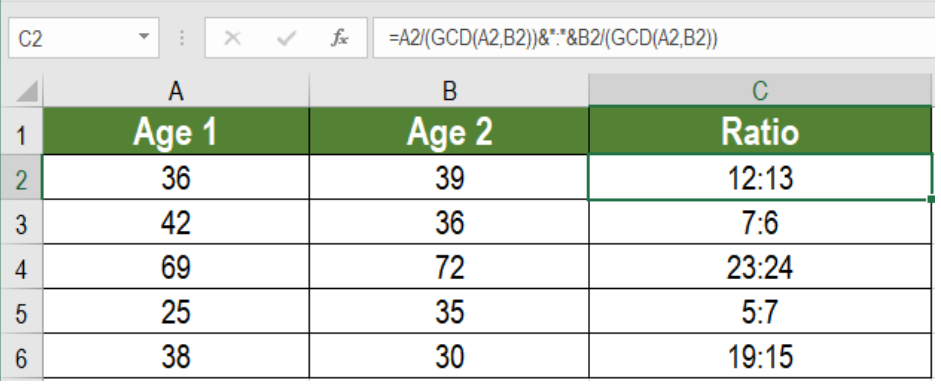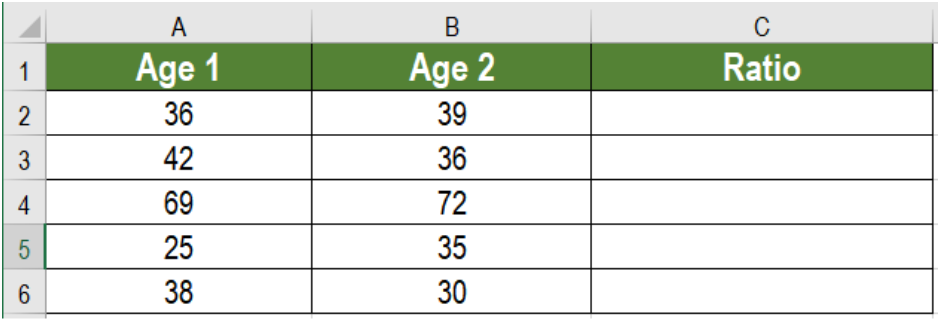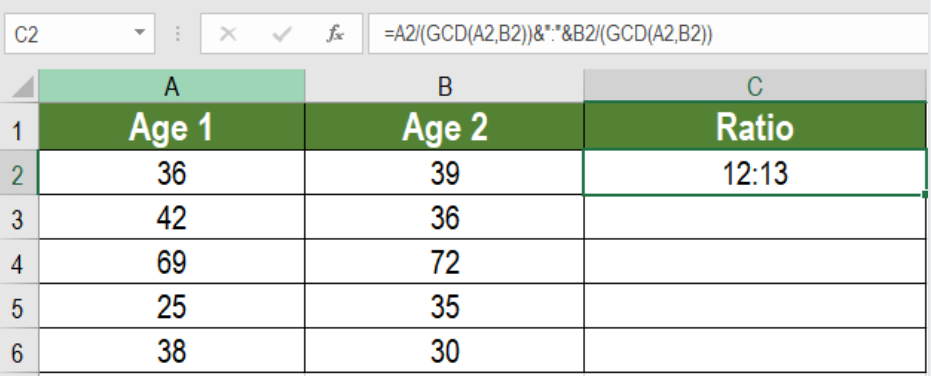Get instant live expert help with Excel or Google Sheets“My Excelchat expert helped me in less than 20 minutes, saving me what would have been 5 hours of work!”

#### Post your problem and you'll get expert help in seconds

Your message must be at least 40 characters
Our professional experts are available now. Your privacy is guaranteed.

# Calculate a ratio from two numbers

Ratio is a way of comparing or relating one thing with other. Finding the ratio between two numbers come handy in many aspects of data analysis. We can use the GCD function in Excel to find the ratio between two numbers. In this tutorial, we will learn how to find the ration from two numbers.Figure 1. Example of How to Find Ratio From Two Numbers

## Syntax

`=num1/GCD(num1,num2)&":"&num2/GCD(num1,num2)`

## How this Formula Works

This formula is based on the GCD function. The two numbers are represented as num1 and num2. Here, we need to calculate the GCD of the two numbers. First, we divide the first number with the GCD. We repeat the same thing with the second number. Lastly, we join them with the concatenate operator (“&”). We use the colon (“:”) to separate the result of the two GCD results.

## Setting up Data

The following example contains a spouse age database. Column A and B contains the ages for both the spouses.Figure 2. The Data Set

To show the ratio between the ages in column D:

• We need to go to cell C2.
• Apply the formula `=A2/(GCD(A2,B2))&":"&B2/GCD(A2,B2) `to C2.
• Press Enter.Figure 3. Applying the Formula

• Drag the formula using the fill handle in the bottom right from cells C2 to C6.

This will show the ratios in column C.

Most of the time, the problem you will need to solve will be more complex than a simple application of a formula or function. If you want to save hours of research and frustration, try our live Excelchat service! Our Excel Experts are available 24/7 to answer any Excel question you may have. We guarantee a connection within 30 seconds and a customized solution within 20 minutes.

### Did this post not answer your question? Get a solution from connecting with the expert.Another blog reader asked this question today on Excelchat:## Subscribe to Excelchat.coAnother blog reader asked this question today on Excelchat: